# Newspaper scraping using Python and News API

• Difficulty Level : Medium
• Last Updated : 29 Dec, 2020

There are mainly two ways to extract data from a website:

• Use the API of the website (if it exists). For example, Facebook has the Facebook Graph API which allows retrieval of data posted on Facebook.
• Access the HTML of the webpage and extract useful information/data from it. This technique is called web scraping or web harvesting or web data extraction.

In this article, we will be using the API of `newsapi`. You can create your own API key by clicking here.

Examples: Let’s determine the concern of a personality like states president cited by newspapers, let’s take the case of MERKEL

 `import` `pprint``import` `requests`` ` ` ` `secret ``=` `"Your API"``  ` `# Define the endpoint``url ``=` `'https://newsapi.org/v2/everything?'``  ` `# Specify the query and``# number of returns``parameters ``=` `{``    ``'q'``: ``'merkel'``, ``# query phrase``    ``'pageSize'``: ``100``,  ``# maximum is 100``    ``'apiKey'``: secret ``# your own API key``}``  ` `# Make the request``response ``=` `requests.get(url, ``                        ``params ``=` `parameters)``  ` `# Convert the response to ``# JSON format and pretty print it``response_json ``=` `response.json()``pprint.pprint(response_json)`

Output: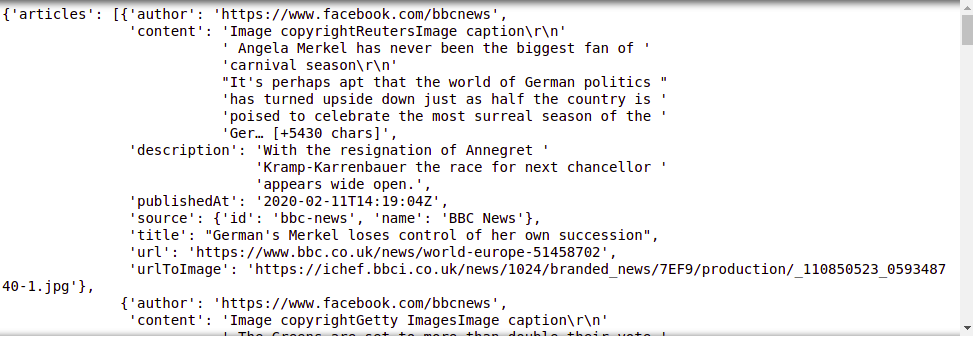Let’s combine all texts and sort the words from the greatest number to lower.

 `from` `wordcloud ``import` `WordCloud``import` `matplotlib.pyplot as plt`` ` ` ` `text_combined ``=` `''`` ` `for` `i ``in` `response_json[``'articles'``]:``     ` `    ``if` `i[``'description'``] !``=` `None``:``        ``text_combined ``+``=` `i[``'description'``] ``+` `' '``         ` `wordcount``=``{}``for` `word ``in` `text_combined.split():``    ``if` `word ``not` `in` `wordcount:``        ``wordcount[word] ``=` `1``    ``else``:``        ``wordcount[word] ``+``=` `1`` ` `for` `k,v, ``in` `sorted``(wordcount.items(),``                   ``key``=``lambda` `words: words[``1``], ``                   ``reverse ``=` `True``):``    ``print``(k,v)`

Output: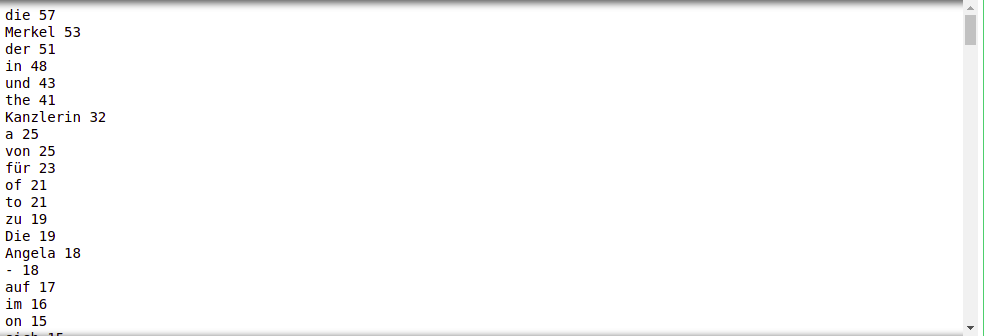This evaluation is ambiguous, we can make it more clear if we delete bad or usless words. Let’s define some of bad_words shown below

bad_words = [“a”, “the”, “of”, “in”, “to”, “and”, “on”, “de”, “with”,
“by”, “at”, “dans”, “ont”, “été”, “les”, “des”, “au”, “et”,
“après”, “avec”, “qui”, “par”, “leurs”, “ils”, “a”, “pour”,
“les”, “on”, “as”, “france”, “eux”, “où”, “son”, “le”, “la”,
“en”, “with”, “is”, “has”, “for”, “that”, “an”, “but”, “be”,
“are”, “du”, “it”, “à”, “had”, “ist”, “Der”, “um”, “zu”, “den”,
“der”, “-“, “und”, “für”, “Die”, “von”, “als”,
“sich”, “nicht”, “nach”, “auch” ]

Now we can delete and format the text by deleting bad words

 `# initializing bad_chars_list ``bad_words ``=` `[``"a"``, ``"the"` `, ``"of"``, ``"in"``, ``"to"``, ``"and"``, ``"on"``, ``"de"``, ``"with"``, ``             ``"by"``, ``"at"``, ``"dans"``, ``"ont"``, ``"été"``, ``"les"``, ``"des"``, ``"au"``, ``"et"``, ``             ``"après"``, ``"avec"``, ``"qui"``, ``"par"``, ``"leurs"``, ``"ils"``, ``"a"``, ``"pour"``, ``             ``"les"``, ``"on"``, ``"as"``, ``"france"``, ``"eux"``, ``"où"``, ``"son"``, ``"le"``, ``"la"``,``             ``"en"``, ``"with"``, ``"is"``, ``"has"``, ``"for"``, ``"that"``, ``"an"``, ``"but"``, ``"be"``, ``             ``"are"``, ``"du"``, ``"it"``, ``"à"``, ``"had"``, ``"ist"``, ``"Der"``, ``"um"``, ``"zu"``, ``"den"``, ``             ``"der"``, ``"-"``, ``"und"``, ``"für"``, ``"Die"``, ``"von"``, ``"als"``,``             ``"sich"``, ``"nicht"``, ``"nach"``, ``"auch"`  `] `` ` ` ` `r ``=` `text_combined.replace(``'\s+'``,``                          ``' '``).replace(``','``, ``                                       ``' '``).replace(``'.'``,``                                                    ``' '``)``words ``=` `r.split()``rst ``=` `[word ``for` `word ``in` `words ``if` `       ``( word.lower() ``not` `in` `bad_words ``        ``and` `len``(word) > ``3``) ]`` ` `rst ``=` `' '``.join(rst)``  ` `wordcount``=``{}`` ` `for` `word ``in` `rst.split():``     ` `    ``if` `word ``not` `in` `wordcount:``        ``wordcount[word] ``=` `1``    ``else``:``        ``wordcount[word] ``+``=` `1``  ` `for` `k,v, ``in` `sorted``(wordcount.items(),``                   ``key``=``lambda` `words: words[``1``],``                   ``reverse ``=` `True``):``    ``print``(k,v)`

Output: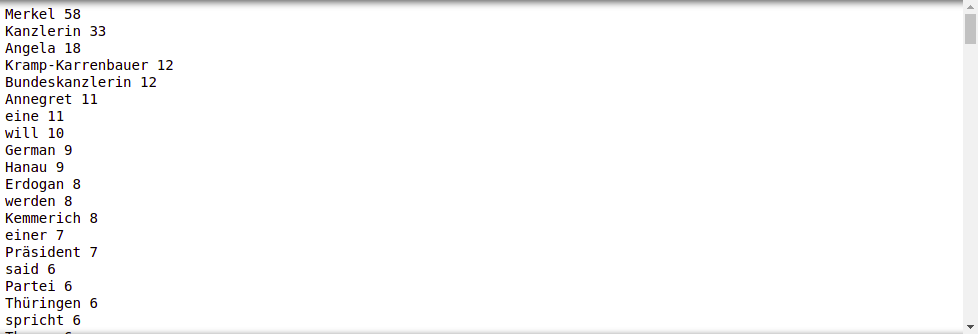Let’s plot the output

 `word ``=` `WordCloud(max_font_size ``=` `40``).generate(rst)``plt.figure()``plt.imshow(word, interpolation ``=``"bilinear"``)``plt.axis(``"off"``)``plt.show()`

Output: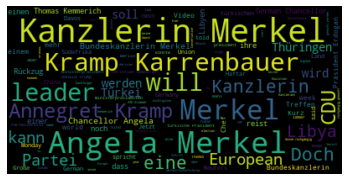As you see in the descriptions of articles, the most dominant concern with Merkel is his defense minister Kramp-Karrenbauer, Kanzlerin just means female chancellor. We can do the same work using titles only

 `title_combined ``=` `''`` ` `for` `i ``in` `response_json[``'articles'``]:``    ``title_combined ``+``=` `i[``'title'``] ``+` `' '``     ` `titles ``=` `title_combined.replace(``'\s+'``,``                                ``' '``).replace(``','``,``                                             ``' '``).replace(``'.'``,``                                                          ``' '``)``words_t ``=` `titles.split()``result ``=` `[word ``for` `word ``in` `words_t ``if``          ``( word.lower() ``not` `in` `bad_words ``and``           ``len``(word) > ``3``) ]`` ` `result ``=` `' '``.join(result)``  ` `wordcount``=``{}`` ` `for` `word ``in` `result.split():``     ` `    ``if` `word ``not` `in` `wordcount:``        ``wordcount[word] ``=` `1``    ``else``:``        ``wordcount[word] ``+``=` `1`` ` `word ``=` `WordCloud(max_font_size``=``40``).generate(result)``plt.figure()``plt.imshow(word, interpolation``=``"bilinear"``)``plt.axis(``"off"``)``plt.show()`

Output: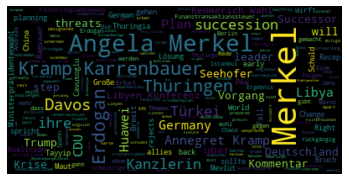From titles, we found out that the most concern with Merkel is Ardogan, turkey president.

My Personal Notes arrow_drop_up